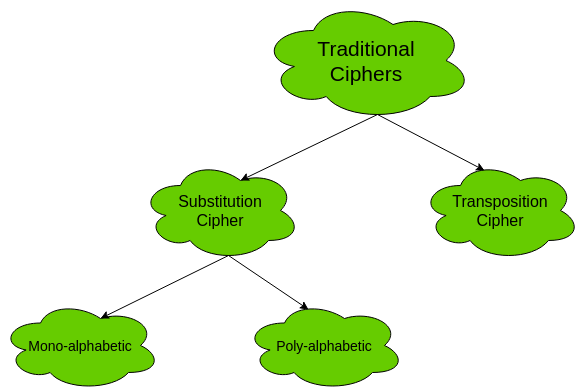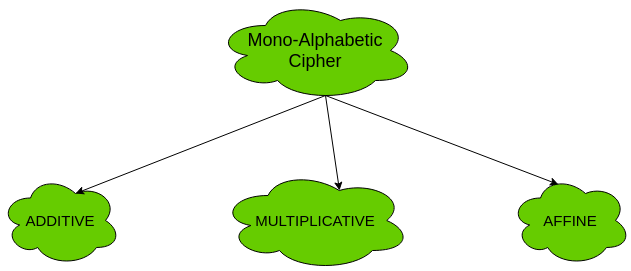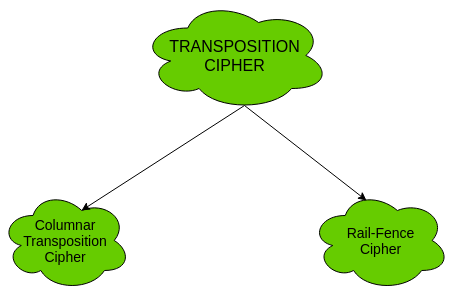• Difficulty Level : Easy
• Last Updated : 14 Oct, 2019

The two types of traditional symmetric ciphers are Substitution Cipher and Transposition Cipher. The following flowchart categories the traditional ciphers:1. Substitution Cipher:
Substitution Ciphers are further divided into Mono-alphabetic Cipher and Poly-alphabetic Cipher.

First, let’s study about mono-alphabetic cipher.

1. Mono-alphabetic Cipher –
In mono-alphabetic ciphers, each symbol in plain-text (eg; ‘o’ in ‘follow’) is mapped to one cipher-text symbol. No matter how many times a symbol occurs in the plain-text, it will correspond to the same cipher-text symbol. For example, if the plain-text is ‘follow’ and the mapping is :

• f -> g
• o -> p
• l -> m
• w -> x

The cipher-text is ‘gpmmpx’.

Types of mono-alphabetic ciphers are:(a). Additive Cipher (Shift Cipher / Caesar Cipher) –
The simplest mono-alphabetic cipher is additive cipher. It is also referred to as ‘Shift Cipher’ or ‘Caesar Cipher’. As the name suggests, ‘addition modulus 2’ operation is performed on the plain-text to obtain a cipher-text.

C = (M + k) mod n
M = (C – k) mod n

where,
C -> cipher-text
M -> message/plain-text
k -> key

The key space is 26. Thus, it is not very secure. It can be broken by brute-force attack.

(b). Multiplicative Cipher –
The multiplicative cipher is similar to additive cipher except the fact that the key bit is multiplied to the plain-text symbol during encryption. Likewise, the cipher-text is multiplied by the multiplicative inverse of key for decryption to obtain back the plain-text.

C = (M * k) mod n
M = (C * k-1) mod n

where,
k-1 -> multiplicative inverse of k (key)

The key space of multiplicative cipher is 12. Thus, it is also not very secure.

(c). Affine Cipher –
The affine cipher is a combination of additive cipher and multiplicative cipher. The key space is 26 * 12 (key space of additive * key space of multiplicative) i.e. 312. It is relatively secure than the above two as the key space is larger.
Here two keys k1 and k2 are used.

C = [(M * k1) + k2] mod n
M = [(C – k2) * k1-1 ] mod n

Now, let’s study about poly-alphabetic cipher.

2. Poly-alphabetic Cipher –
In poly-alphabetic ciphers, every symbol in plain-text is mapped to a different cipher-text symbol regardless of its occurrence. Every different occurrence of a symbol has different mapping to a cipher-text. For example, in the plain-text ‘follow’, the mapping is :

f -> q
o -> w
l -> e
l -> r
o -> t
w -> y

Thus, the cipher text is ‘qwerty’.

Types of poly-alphabetic ciphers are:2. Transposition Cipher:
The transposition cipher does not deal with substitution of one symbol with another. It focuses on changing the position of the symbol in the plain-text. A symbol in the first position in plain-text may occur in fifth position in cipher-text.

Two of the transposition ciphers are:1. Columnar Transposition Cipher –
For information and implementation, see Columnar Transposition Cipher
2. Rail-Fence Cipher –
For information and implementation, see Rail-Fence Cipher
My Personal Notes arrow_drop_up